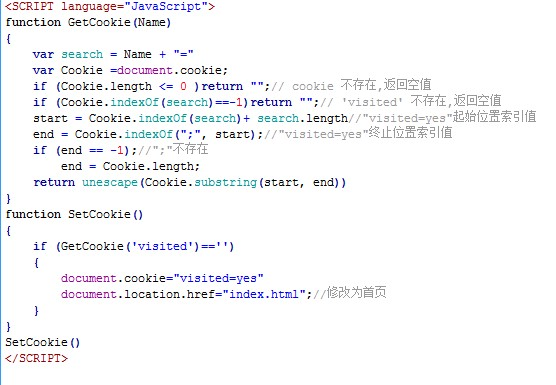/ 猿问

2019-03-20 14:13:53

<SCRIPT language="JavaScript">
{
var search = Name + "="
if (end == -1);//";"不存在
}
{
{
document.location.href="index.html";//修改为首页
}
}
</SCRIPT>

•## 3 回答123 var d= new Date();d.setHours(d.getHours() + (24 * 30)); //保存一个月document.cookie = "visited=yes; expires=" + d.toGMTString();qq_笑_17

 1234567891011121314151617181920212223242526272829303132 var cookie = function () {        //expires参数是Date()实例        var set = function (cookieName, cookieValue, expires, path, domain, secure) {            var A = arguments;            var H = arguments.length;            var B = (2 < H) ? A.toGMTString() : "";            var G = (3 < H) ? A : "";            var D = (4 < H) ? A : "";            var F = (5 < H) ? A : false;            document.cookie = cookieName + "=" + escape(cookieValue) + ";expires =" + B + ";path = " + G + ";domain =" + D + ((F == true) ? ";secure" : " ")        }        //cname:cookie名称，itemName:该cookie中某一项名称        var get = function (cname, itemName) {            var A = document.cookie.match(new RegExp("(^| )" + cname + "=([^;]*)(;|\$)"));            if (A) {                if (itemName) {                    A = A.match(new RegExp("(?:^|&)" + itemName + "=([^&]*)(?:&|\$)"))                    return A ? A : "";                }                else {                    return A;                }            }            else {                return "";            }        }        return {            set: set,            get: get        }    }();

//使用：setDate(exdate.getDate()+10) //getDate()表示天数，这里表示存储10天
setDate(exdate.getHours()+10) //存储10个小时
...

• 3 回答
• 0 关注
• 826 浏览

0/150